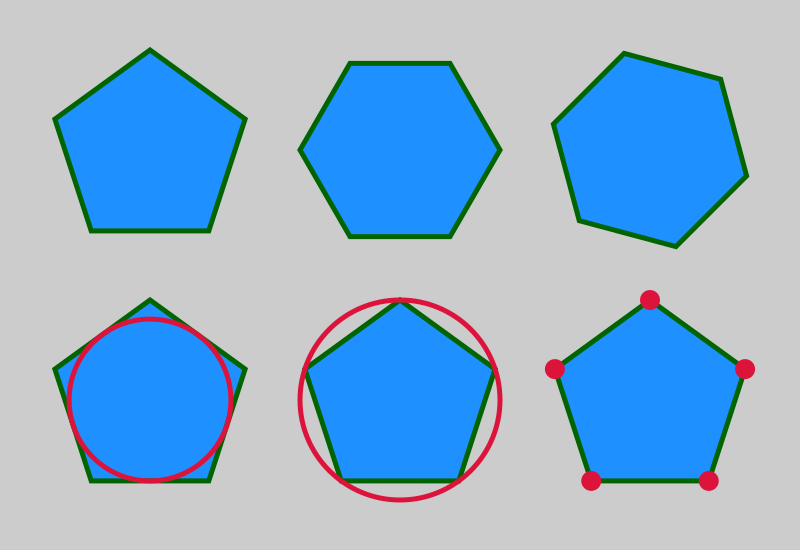# Regular polygons in generativepy

Martin McBride, 2022-06-05
Tags generativepy tutorial fill stroke polygon regular polygon
Categories generativepy generativepy tutorialThis tutorial shows how to create regular polygons in generativepy, using the RegularPolygon class.

Here are some related tutorials that contain useful information about other features this tutorial uses:

## RegularPolygon example code

Here is the code to show some of the features of RegularPolygon:

from generativepy.drawing import make_image, setup
from generativepy.color import Color
from generativepy.geometry import RegularPolygon, Circle
import math

def draw(ctx, pixel_width, pixel_height, frame_no, frame_count):

setup(ctx, pixel_width, pixel_height, background=Color(0.8))

red = Color('crimson')
green = Color('darkgreen')
blue = Color('dodgerblue')

.fill(blue)\
.stroke(green, 5)

.fill(blue)\
.stroke(green, 5)

.fill(blue)\
.stroke(green, 5)

p = RegularPolygon(ctx).of_centre_sides_radius((150, 400), 5, 100)\
.fill(blue)\
.stroke(green, 5)

p = RegularPolygon(ctx).of_centre_sides_radius((400, 400), 5, 100)\
.fill(blue)\
.stroke(green, 5)

p = RegularPolygon(ctx).of_centre_sides_radius((650, 400), 5, 100)\
.fill(blue)\
.stroke(green, 5)
for v in p.vertices:

make_image("regularpolygons-tutorial.png", draw, 800, 550)


This code is available on github in tutorial/shapes/regularpolygons.py.

Here is the resulting image:We will examine this code in the sections below.

## Drawing basic regular polygons

This section of the code draws the two polygons in the top left of the image:

    RegularPolygon(ctx).of_centre_sides_radius((150, 150), 5, 100)\
.fill(blue)\
.stroke(green, 5)

.fill(blue)\
.stroke(green, 5)


This code creates two polygons with:

• A suitable centre point to position them on the page.
• 5 sides (pentagon) and 6 sides (hexagon).
• Radius of 100 to set the size.
• Filled in blue and outlined in green.

Notice that both shapes have horizontal bases.

## Drawing a rotated polygon

This code draws the hexagon in the top left of the image:

    RegularPolygon(ctx).of_centre_sides_radius((650, 150), 6, 100, math.pi/12)\
.fill(blue)\
.stroke(green, 5)


This is drawn in the same way as the previous hexagon, but with an angle of math.pi/12 radians (15 degrees). This rotates the shape 15 degrees clockwise about its centre, so the base is no longer horizontal.

## Drawing an inner circle

This code draws the same pentagon as before, but with an inner circle (bottom left of the main image):

    p = RegularPolygon(ctx).of_centre_sides_radius((150, 400), 5, 100)\
.fill(blue)\
.stroke(green, 5)


When we draw the pentagon, we also store the RegularPolygon object as p.

We then draw a circle. The circle has the same centre as the polygon, and a radius equal to the inner radius of the polygon. This is obtained from p.inner_radius.

The circle just fits inside the polygon.

## Drawing an outer circle

This code draws the same pentagon as before, but with an outer circle (bottom centre of the main image):

    p = RegularPolygon(ctx).of_centre_sides_radius((400, 400), 5, 100)\
.fill(blue)\
.stroke(green, 5)


This time, the circle has the same centre as the polygon, and a radius equal to the outer radius of the polygon. This is obtained from p.outer_radius.

The polygon just fits inside the circle.

## Drawing the vertices

This code draws the same pentagon as before, but marks each corner with a dot (bottom right of the main image):

    p = RegularPolygon(ctx).of_centre_sides_radius((650, 400), 5, 100)\
.fill(blue)\
.stroke(green, 5)
for v in p.vertices:

Then we use p.vertices to get the corners of the polygon. This returns a tuple of five coordinates (x, y) corresponding to the five vertices of the pentagon.